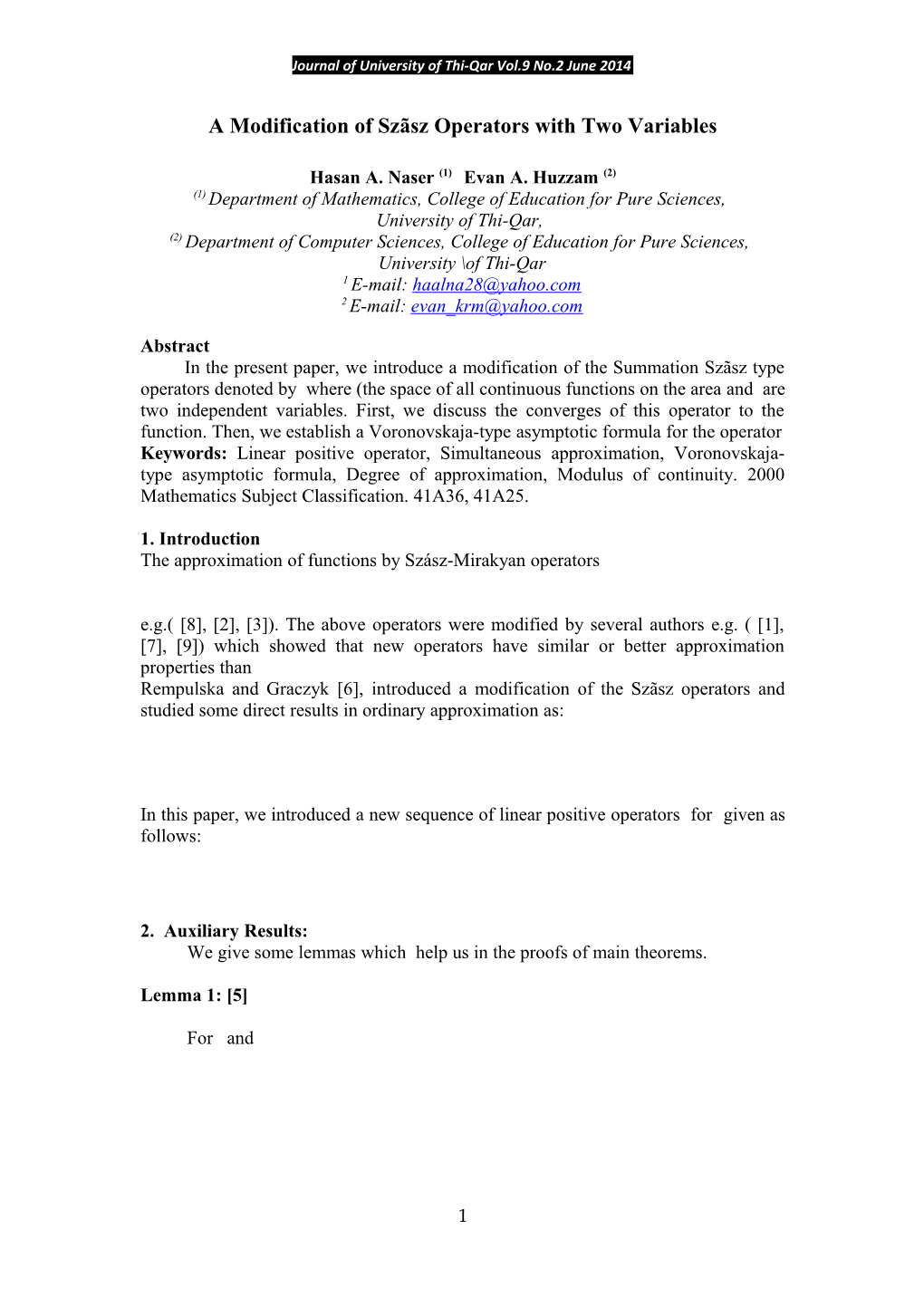# A Modification of Szãsz Operators with Two VariablesJournal of University of Thi-Qar Vol.9 No.2 June 2014

A Modification of Szãsz Operators with Two Variables

Hasan A. Naser(1) Evan A. Huzzam(2)

(1) Department of Mathematics, College of Education for Pure Sciences,

University of Thi-Qar,

(2) Department of Computer Sciences, College of Education for Pure Sciences,

University \of Thi-Qar

1 E-mail:

2 E-mail:

Abstract

In the present paper, we introduce a modification of the Summation Szãsz type operators denoted by where (the space of all continuous functions on the area and are two independent variables. First, we discuss the converges of this operator to the function. Then, we establish a Voronovskaja-type asymptotic formula for the operator

Keywords: Linear positive operator, Simultaneous approximation, Voronovskaja-type asymptotic formula, Degree of approximation, Modulus of continuity. 2000 Mathematics Subject Classification.41A36, 41A25.

1. Introduction

The approximation of functions by Szász-Mirakyan operators

e.g.( , , ). The above operators were modified by several authors e.g. ( , , ) which showed that new operators have similar or better approximation properties than

Rempulska and Graczyk ,introduced a modification of the Szãsz operators and studied some direct results in ordinary approximation as:

In this paper, we introduced a new sequence of linear positive operators for given as follows:

2. Auxiliary Results:

We give some lemmas which help us in the proofs of main theorems.

Lemma 1: 

For and

terms of form where

Definition 1:

A function is of smaller order than function as , if we indicate this by writing .

Lemma 2: 

Forand , we get:

Lemma 3: 

For each and for all we get:

Definition 2:

The norm of the space which is defined as:

Our first theorem shows that the operators converges to the function as .

Theorem 1:

Let . If for all the following conditions hold:

then

Proof:

By using Lemmas 1 and 2, we get:

By the same way we can prove (3), as follows:

Then, by Korovkin's theorem  we get:

Finally, we give a Voronovskaja-type asymptotic formula for the operators .

Theorem 2:

For such that,, suppose that , and exist and are continuous at a point, then:

Proof:

By Taylor's formula for, about the point we have:

whereis function from the space , and as then

Using Lemma 3, we have:

Hence,

To complete the proof, we must show that the term

.

By using Cauchy-Schwartz inequality, we get:

Now, by the properties and Theorem 1, we have:

Then from Lemma 3, we get:

Therefore,

Then, the proof of the Theorem 2 is over.

References

Ciupa, Approximation by a generalized Szász type operator, J. Comput. Anal.andApplic., 5(4) (2003), 413–424.

Devore and Lorentz, Constructive Approximation , Springer Verlag, Berlin, New York, 1993.

 Ditzian and Totik, Moduli of smoothness , Springer-Verlag, New-York, 1987.

P.P. Korovkin: Linear Operators and Approximation Theory, Hindustan publ. Corp. Delhi, 1960 (Translated from Russian Edition) (1959).

H. A. Naser. " On Generalization for Some Szãsz Type Operators " M.SC. thesis , University of Basrah, 2011.

Rempulska and Graczyk, Approximation by modified SzãszMirakyan operator, J. Inequal. Pure and Appl. Math., 10(3)(2009), Art. 61, 1-8.

Rempulska and Walczak. Modified Szãsz-Mirakyan operators.Math.Balk., New Ser. 18 (2004), 53-63.

Szãsz, Generalization of S. Bernstein's polynomials to the infinite interval, J. Res. Nat. Bur. Standard, 45(1950), 239-245.

Walczak, On the convergence of the modified Szász-Mirakyan operators, Yokohama Math. J., 51(1) (2004), 11–18.

الخلاصة

في هذا البحث, نقدم تحسين للمؤثر من النمط مجموع Szãszونرمز له , حيث أن الدالة والمتغيرين يكونان مستقلان. أولا, نناقش تقارب المؤثر إلى الدالة وبعد ذلك نثبت صيغة ڤرونوڤسكي للتقارب (Voronovskaja-type asymptotic formula) للمؤثر

1